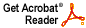# The bounded weak star topology and the general strict topology

Joel H. Shapiro

J. Functional analysis 8 (1971), 275--286

Abstract: Suppose that B is a Banach algebra with bounded approximate identity, and that X is a is a left Banach module over B. The strict topology on X is the topology generated by the set {p_b: b in B} of semi-norms, where p_b(x) = ||bx || for b in B, x in X. The original strict topology was introduced by R. C. Buck [Michigan Math. J. 5 (1958), 95--104] and the above generalization is due to F. D. Sentilles and D. C. Taylor [Trans. Amer. Math. Soc. 142 (1969), 141 152]. In the present paper, results of L. A. Rubel and J. V. Ryff [J. Functional Analysis 5 (1970), 167 --183] and myself [Trans. Amer. Math. Soc. 157 (1971), 471 479] are extended to this version of the strict topology. Let M be the set of strictly continuous bounded linear functionals on X, and, for E a subspace of X, let E^o be the annihilator of E. The main result states that if E is a subspace of X with strictly compact unit ball then the natural mapping f from E onto the quotient space (M/E^o) is a linear isometry, and the strict topology is the bounded weak star topology (the strongest topology that agrees with the weak star topology on bounded sets) of E. Using this result I give explicit seminorms of the bounded weak star topology on a space with a boundedly complete basis. A final result shows that, if G is a locally compact abelian group with Haar measure, then G is compact if and only if the bounded weak star topology induced in L p(G) (1 < p <=infinity) by Lq(G) (1/q +1/p = 1) is the strict topology induced by L^1(G). From this it follows that, in its strict topology, the Banach space of bounded analytic functions on the unit disc is a topological algebra under convolution.
Download .pdf file (444KB)